# Memory leak or just calculus?

04.24.17This post was originally posted on 23 May, 2016. It followed our engineers as they found what appeared to be a memory leak, and then resorted to calculus to convince themselves that what they were observing was a consequence of the probability distribution of their randomised algorithm.

Making distributed systems is hard. At Improbable, we like it when our system works, and it makes us sad when it breaks. For this reason, we have a number of test simulations we deploy regularly on our cloud infrastructure and comprehensively measure, to give us some degree of confidence that everything is working properly and we haven’t introduced performance or correctness regressions.

Recently, one of our engineers noticed something worrying: one of our test deployments had been running for several hours, and the graph tracking this particular deployment’s memory usage was going up and to the right. The increase in memory usage was slow, but unmistakable: it looked pretty obviously like a memory leak . In a system like SpatialOS that has to run for a long time without failure or loss of stability, any sort of memory leak, even a very small one, is certainly cause for concern. Worse, bugs like these can be quite tricky to investigate and sort out.

However, after studying our metrics more closely we noticed something odd. The offending graph was an aggregation over a metric that tracked memory usage in a spatial way. That is, we were looking at a graph of the maximum memory usage per unit of space over various sub-regions in the world. Drilling down, we saw that the so-called memory leak did not manifest uniformly throughout the world: in fact, there was a conspicuous pattern. The severity of the memory leak in a given sub-region was strongly correlated with the relative location in space of that sub-region. We observed that memory usage was more-or-less stable toward the edges of the world, and only began to trend upwards as we considered sub-regions closer to the centre of the world.

## Test players

To understand what was going on, it’s necessary explain what our test simulation actually does. It’s designed to look a bit like a simplified version of any open-world online game: we have a large world, filled with a thousand or so player entities controlled by dummy clients. These dummy clients connect from outside our cloud and, to SpatialOS, are indistinguishable from real clients controlled by actual humans—but they are automated. These “test players” are supposed to be reasonable simulacra of genuine players: they wander non-deterministically through the world, can observe each other, and SpatialOS has to carry out all the same data synchronisation work that would be necessary if they were real players.

What’s important is exactly how these mock-players navigate the world. They start out distributed randomly throughout the world, and their subsequent behaviour is governed by the following steps:

1. Let the starting point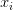$\xi)$ be the player’s current position in the world.
2. Pick a destination point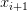$\xi+1)$ uniformly at random in the world.
3. Proceed from$\xi)$ to$\xi+1)$ at a fixed speed.
4. Upon reaching$\xi+1)$, go back to step 1.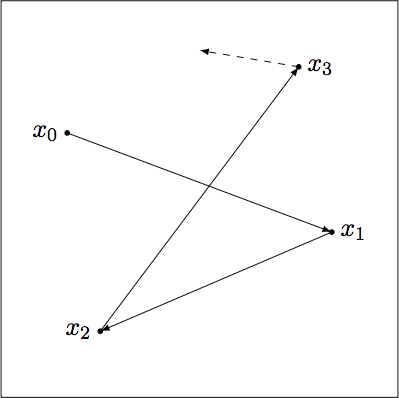Assuming this process repeats forever: on average, where can we expect the players to end up? That is, sampling a player’s position at an arbitrary time gives rise to some probability distribution over the world itself—what does this distribution look like?

Our thought process was this: intuitively, it seems like the distribution should be weighted more heavily towards the centre of the world. After all, there are many possible routes a player might take through the middle of the world, but far fewer through points on the edge. If this is correct, then the upwards trend in memory usage could be explained simply by the fact that the thousand players start out distributed uniformly (so no matter where one looks in the world, there is a comparable amount of work to be done) but tend towards this limiting, centre-heavy distribution (so regions of space towards the centre of the world eventually become disproportionately complex, and require more resources to simulate).

Intuitive reasoning is all well and good, but to be sure we need something more rigorous.

## Calculus

In the case of our test simulation, the world is a (large) three-dimensional cube, but for simplicity’s sake we analyse the case where the world is the unit interval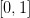$\[0,1])$.

A player’s trajectory through the world can be viewed as an (infinite) sequence of path segments, where each path segment has a pair of endpoints ($\xi)$ and$\xi+1)$) and is traversed in time proportional to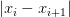$\|xi-xi+1|)$. The probability distribution of a player’s position in the world at some arbitrary time can be expressed as a probability density function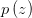$\p(z))$, where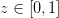$\z E [0,1])$ represents a position in the world. This can be computed by summing the contribution from each possible path segment, weighted by the likelihood that a player is currently traversing that path segment: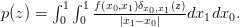$\p(z)= integral)$

Here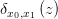$\deltax0x1(z))$ is 1 if$\z)$ lies on the path segment from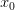$\x0)$ to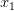$\delta_{x_0,x_1}\left(z\right)$ (i.e.\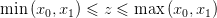) and 0 otherwise, and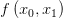$\fx0x1)$ is the probability density function giving the distribution for which path segment is currently being traversed at an arbitrary time. This can be read as combination of probability density functions:$\fx0x1)$ means “given we are on the way from$\x0)$ to$\delta_{x_0,x_1}\left(z\right)$”, and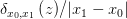$\delta_{x_0,x_1}\left(z\right)$ is the probability density function for a single traversal between$\x0)$ and$\delta_{x_0,x_1}\left(z\right)$.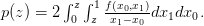$\delta_{x_0,x_1}\left(z\right)$

Now, path segments are chosen uniformly at random at each endpoint, but since the player takes a longer time to traverse longer path segments, the likelihood of a path segment being the current one at an arbitary time is proportional the length of the segment. Therefore, with normalisation,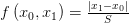$\f(x0,x1) =)$

with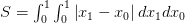$\S = integral)$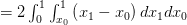$\= 2 integral)$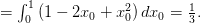$\= integral)$

Cancelling, we have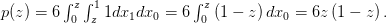$\p(z) = 6(integral) = 6z(1-z))$

Note that this has all the properties we expect: it’s symmetric around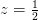$\z=1/2/)$, positive on$\[0,1])$, and its integral over the whole world is 1. That is,$\p(z))$ really is a probability density function, and the quadratic distribution it describes is a plausible explanation for our “memory leak”. Better still, we verified this result empirically with a simple one-dimensional simulation of our test players’ behaviour (a simulation of a simulation, if you will).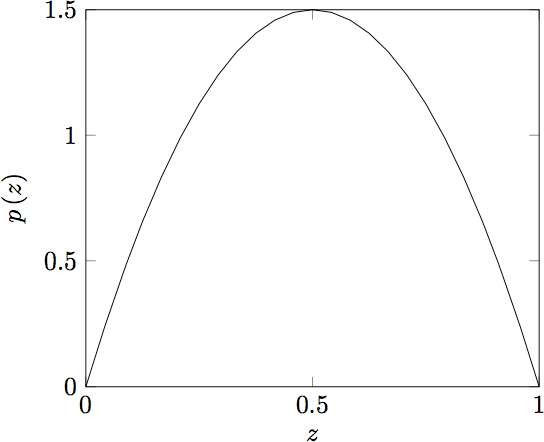## Resolution

We now have an explanation, but the situation isn’t ideal. What if this odd evolution in the test simulation’s memory usage as the test players slowly congregate toward the centre of the world hides a real memory leak?

One obvious solution, now that we have this formula, is to initially distribute the players in the world according to$\p(z))$ (or a higher-dimension generalisation)—then the simulation will be steady-state, at least.

On the other hand, it could cause some confusion if different regions in the test deployment have wildly different resource requirements. To avoid this, it might be even better if we could somehow modify the test players’ behaviour such that they maintain a consistent uniform distribution despite their random movement. One might suppose that we could weight each choice of destination point away from the middle in order to cancel out$\p(z))$  and obtain a uniform distribution. Unfortunately, this does not seem to be possible: doing so would amount to finding a density function g with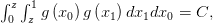$\integral = C)$

where C is some constant. Letting G be the antiderivative of g with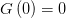$\G(0)=0)$ and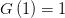$\G(1)=1)$, the left-hand side is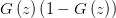$\G(z)(1-G(z)))$. But  is a quadratic equation and so has at most two solutions; so G is a step function and g must be zero almost everywhere.

By analogy, even in the discrete version (choosing from only n possible destination points spaced evenly along the unit interval) there is no way to obtain a uniform distribution except in the trivial cases where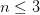$\n<=3)$.

The only practical way to do fix the problem, then, is to use a qualitatively different process for controlling the players’ behaviour: for instance, a random walk on a bounded n-dimensional lattice, which is well-known to converge to a uniform distribution.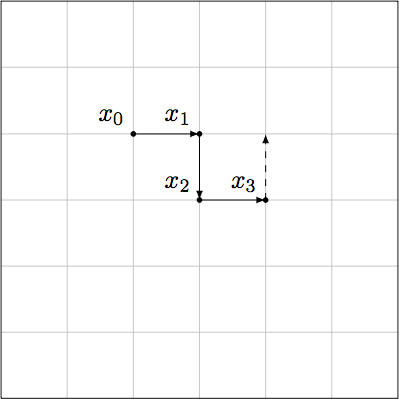A random walk on a bounded 2-dimensional lattice. Points not actually on the grid will never be visited at all, but we can make the grid arbitrarily fine-grained, and distribution over the grid is uniform.

For further info, the complete paper can be found here.

#### Footnotes

1. The SpatialOS kernel runs on the JVM, but this in no way precludes the possibility of memory leaks: for example, perhaps there is some internal data structure that we populate as necessary but (under certain circumstances) neglect to clean up—so it grows without bound over time.

Check out our open job opportunities.